Next: FFTC1: One-Dimensional Complex Fourier Up: FFTEASY C Routines for Previous: Output of Fourier Transforms

# How FFTEASY works

FFTEASY uses a Cooley-Tukey Fast Fourier Transform algorithm. For an excellent discussion of Fourier transforms (continuous and discrete) in general and the Cooley-Tukey algorithm in particular see Numerical Recipes. Here I only briefly describe the algorithm and how it is implemented in FFTEASY.

The heart of the method is the Danielson-Lanczos (DL) formula that allows one to compute a discrete Fourier transform (DFT) of sizeby separately computing two FTs of size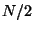. Letbe the DFT of a function. Then letbe the DFT of the even pointsand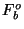be the DFT of the odd points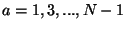. The DL formula then says(9)

For simplicity defineso(10)

Note thatand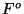both have periodwhile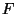has period. This formula can be applied recursively so thatandare each in turn calculated from two DFTs of size. Assuming the originalwas a power of two this process can be repeated all the way down to DFTs of single points, which are just identity operations (i.e.for a single point).

It turns out that rather than keeping track of successive divisions into even and odd points, the data in the original array can be rearranged in such a way thatandare always sequential. In other words once the array has been rearranged in this way the DL formula can be used to combine the first two elements into anDFT, and then combine that DFT with the succeedingDFT into anDFT, and so on. The way to rearrange the array to accomplish this is to arrange it in bit-reverse order. In other words ifthen the four elements of your original array are,,, and. To bit-reverse this array you would swap the middle two elements, leaving the first and last where they are. With some work you should be able to convince yourself that bit-reversal is equivalent to pulling out successive arrays of even and odd points. Otherwise you can take my word for it because I'm not going to prove it to you here.

The following sections describe each of the four FFTEASY functions. Note that all of the functions take as input an array of floats but immediately typecast it as an array of complex numbers, each consisting of two floats representing real and complex parts. So any time I refer to the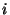th element of an array I mean theth complex element, corresponding to the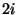andelements of the actual array of floats. Formulas are given here in terms of complex numbers and then implemented in the functions explicitly in terms of real and imaginary parts.

SubsectionsNext: FFTC1: One-Dimensional Complex Fourier Up: FFTEASY C Routines for Previous: Output of Fourier Transforms